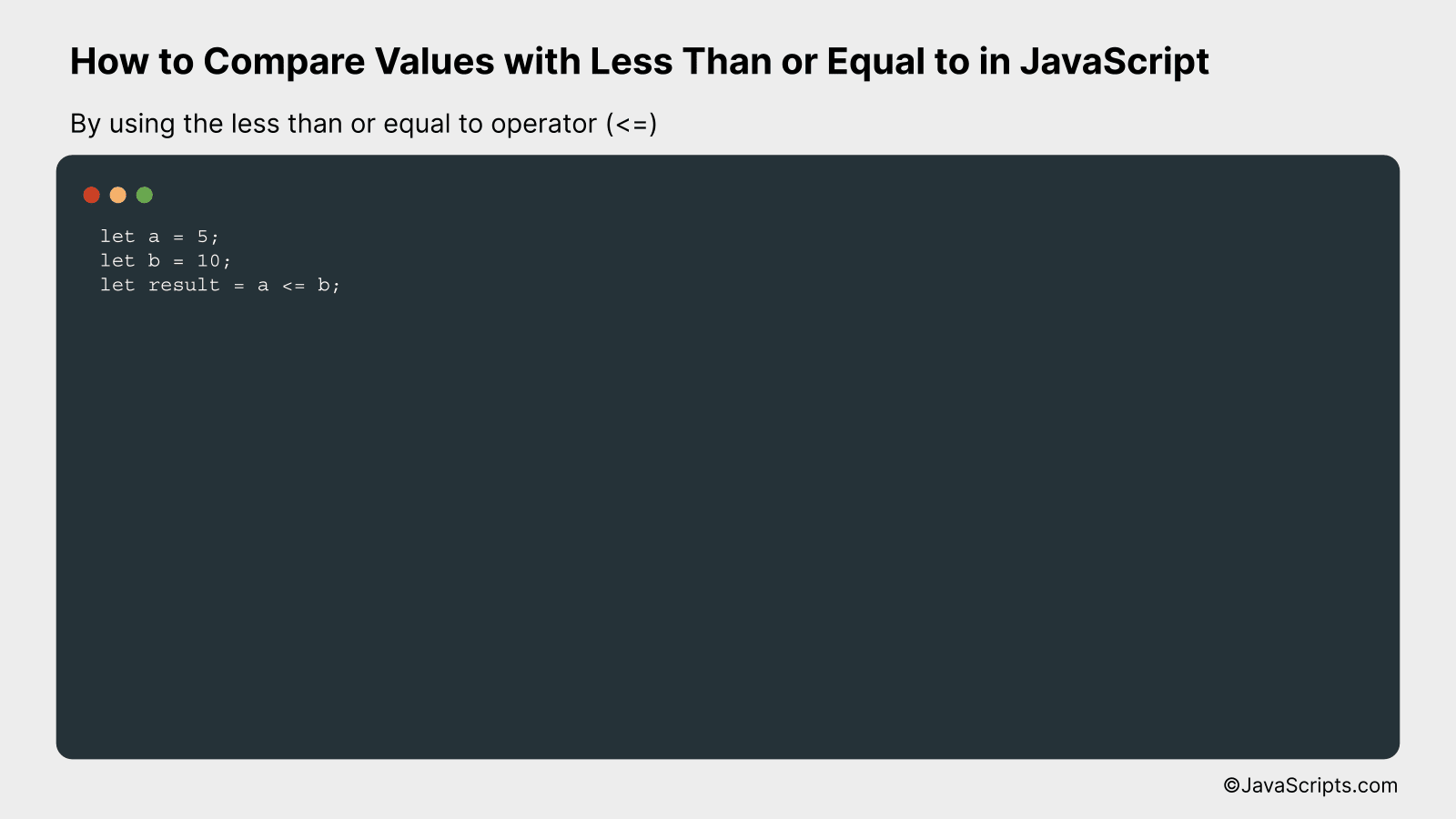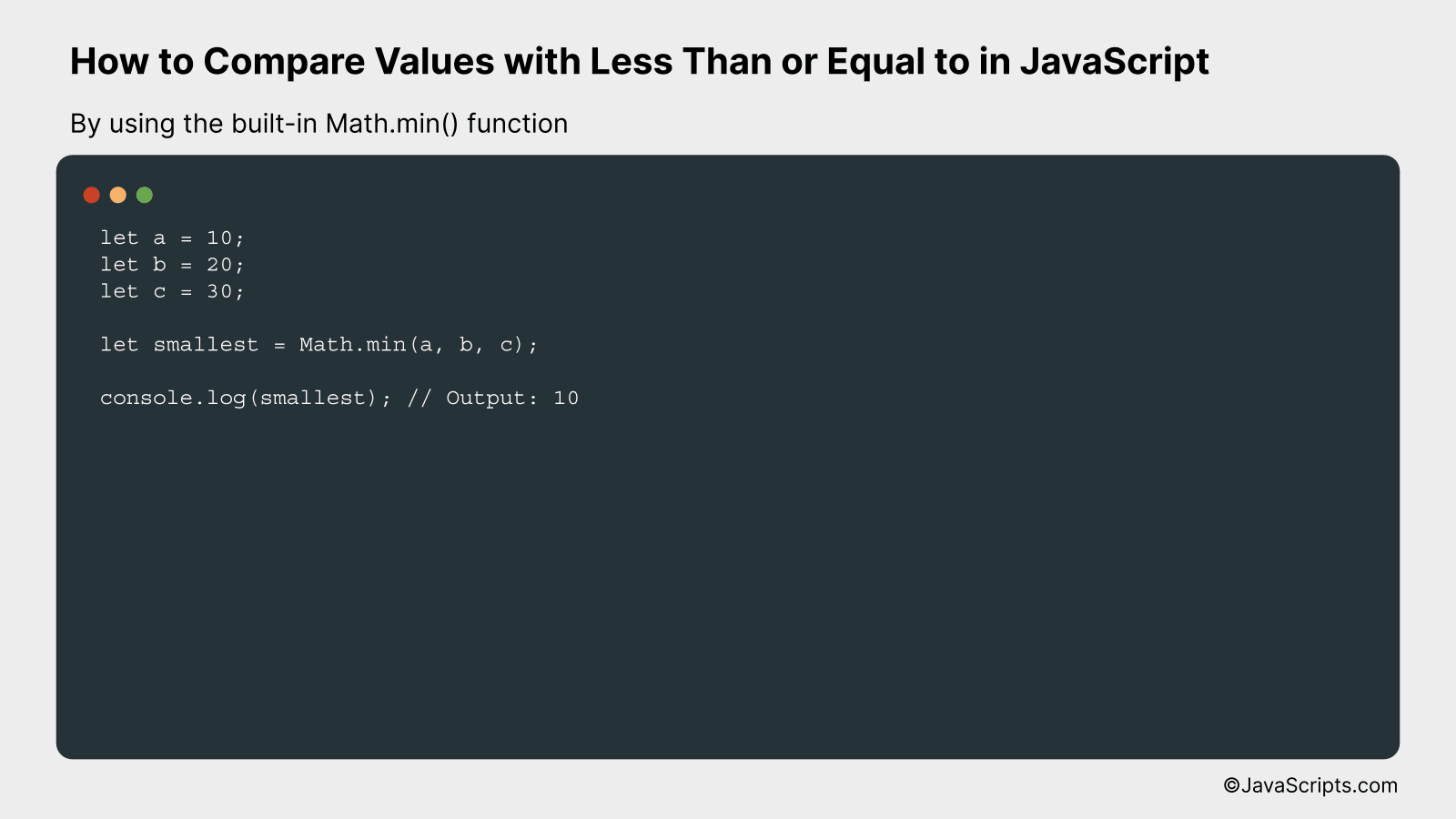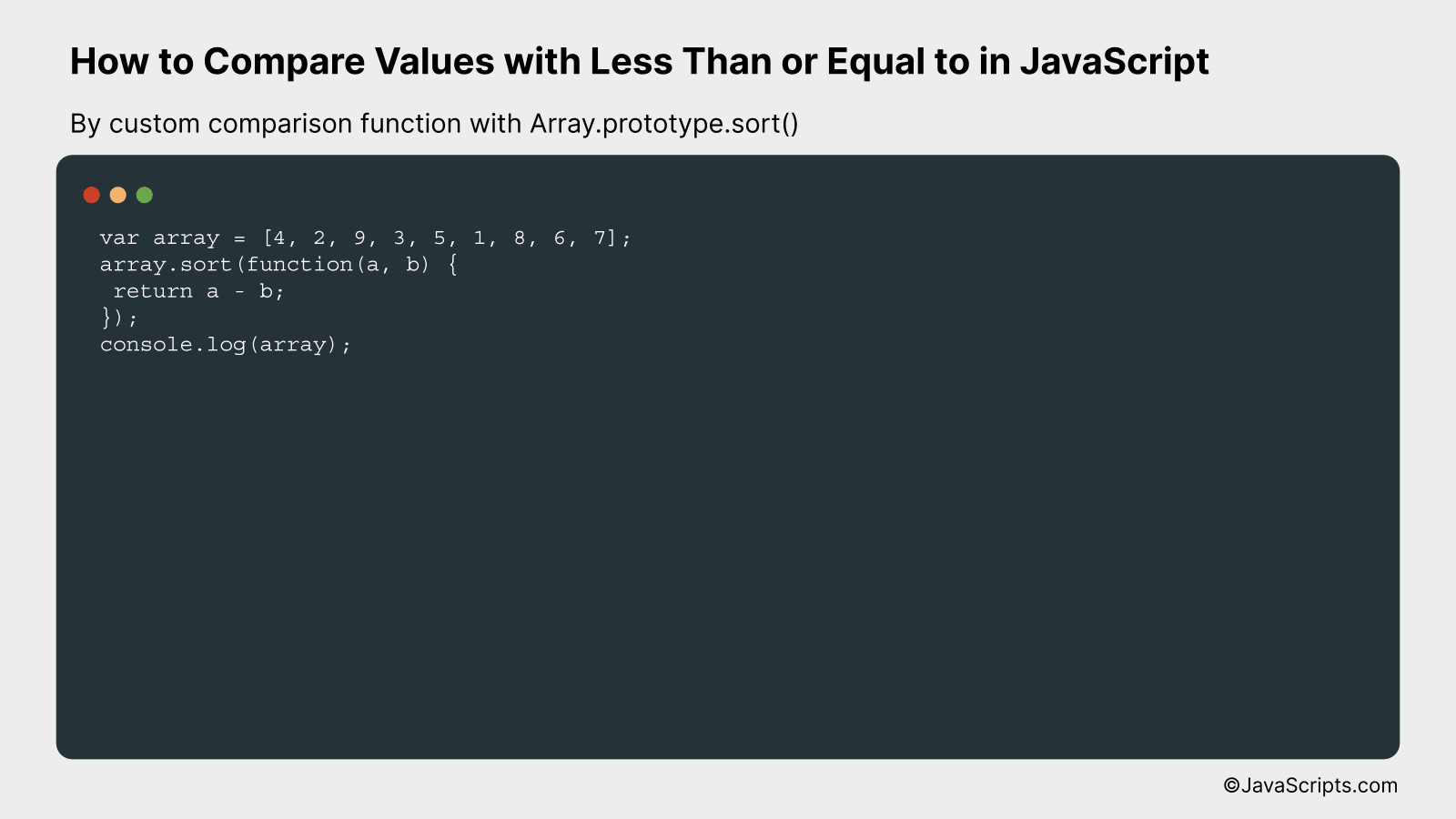# How to Compare Values with Less Than or Equal to in JavaScript

JavaScript forms the backbone of modern web development, helping developers create interactive and dynamic experiences for website users. As one of its vital functions, JavaScript allows for various comparisons between values, a feature we will explore today.

Are you stumped when it comes to using the ‘less than’ or ‘equal to’ operators in JavaScript? Don’t fret, because we’re here to unravel the mystery together. We’ll break down these concepts using easy-to-understand language and examples. Buckle up for an enlightening journey into JavaScript’s comparison operators.

In this post, we will be looking at the following 3 ways to compare values with less than or equal to in JavaScript:

• By using the less than or equal to operator (<=)
• By using the built-in Math.min() function
• By custom comparison function with Array.prototype.sort()

Let’s explore each…

## #1 – By using the less than or equal to operator (<=)The less than or equal to operator (<=) in JavaScript is a comparison operator that checks if the value of the left operand is less than or equal to the value of the right operand. It returns a boolean value, true or false, based on the comparison. Here's an example:

``````
let a = 5;
let b = 10;
let result = a <= b;
``````

### How it works

In the above code, the less than or equal to operator (<=) is used to compare the values of 'a' and 'b'. If 'a' is less than or equal to 'b', then it returns true; otherwise, it returns false.

• First, we initialize two variables 'a' and 'b' with the values 5 and 10 respectively.
• Then, we compare 'a' and 'b' using the less than or equal to operator (<=).
• If the value of 'a' is less than or equal to 'b', the comparison will return true. If not, it returns false.
• In this case, since 5 is indeed less than or equal to 10, the variable 'result' will hold the boolean value 'true'.

## #2 - By using the built-in Math.min() functionThe Math.min() function in JavaScript compares two or more numbers and returns the smallest of them. This function is typically used when you need to find the minimum value among multiple values, which can be better understood through an example.

``````
let a = 10;
let b = 20;
let c = 30;

let smallest = Math.min(a, b, c);

console.log(smallest); // Output: 10
```
```

### How it works

The JavaScript Math.min() function compares all the given parameters and returns the one with the smallest value. If no parameters are provided, it returns infinity.

• Step 1: We declare three variables 'a', 'b', and 'c' with values 10, 20 and 30 respectively.
• Step 2: The Math.min() function is called with 'a', 'b', and 'c' as arguments. This function checks all the input parameters and identifies the smallest one.
• Step 3: The smallest value is stored in a new variable called 'smallest'.
• Step 4: We use console.log() to print the result which is '10' in this case, since 10 is the smallest among the three numbers.

## #3 - By custom comparison function with Array.prototype.sort()We're going to use JavaScript's Array.prototype.sort() function to compare values in an array, employing a custom function that sorts elements using the 'less than or equal to' comparator. Below is an example of how it's done.

``````
var array = [4, 2, 9, 3, 5, 1, 8, 6, 7];
array.sort(function(a, b) {
return a - b;
});
console.log(array);
``````

### How it works

The Array.prototype.sort() function in JavaScript sorts the elements of an array in place and returns the array. The default sort order is built upon string conversion of each element, but a compare function can be provided to define a custom sort order. In this case, we've provided a 'less than or equal to' comparator.

• Firstly, we declare an array with various numeric elements.
• Then, we call the sort() function on the array, which is a method that sorts the elements of an array in place and returns the array.
• The sort function typically sorts elements as strings, but we've provided a comparator function that sorts numerically. This function takes two arguments, a and b, which represent any two elements in the array.
• Inside this function, we're subtracting b from a. If a is less than b, a negative value will be returned, indicating that a should come before b. If a is equal to b, 0 will be returned, indicating no change in order. If a is greater than b, a positive value will be returned, indicating that a should come after b.
• Finally, we print the sorted array to the console. The numbers should now be ordered in ascending order due to our custom comparison function.

Related:

In conclusion, comparing values with 'less than or equal to' in JavaScript is a basic yet powerful tool in coding. Mastering this operator can significantly enhance your coding proficiency.

Remember, practice is key in programming. So, keep experimenting with 'less than or equal to' in different scenarios to solidify your understanding.